#第三次实验报告

----------------周萌  4月21日

### 实验项目

5.3.1 ：用for语句实现循环

5.3.2 ：用while循环语句实现循环

5.3.3 ：用do-while语句实现循环

5.3.4 ：用while和for语句配合实现循环

5.3.5 ：用for语句嵌套实现循环

### 实验内容

?问题描述：编写一程序，求出1，1+2，1+2+3.....数列中前n项的值。

?要求：掌握for语句实现循环的方法。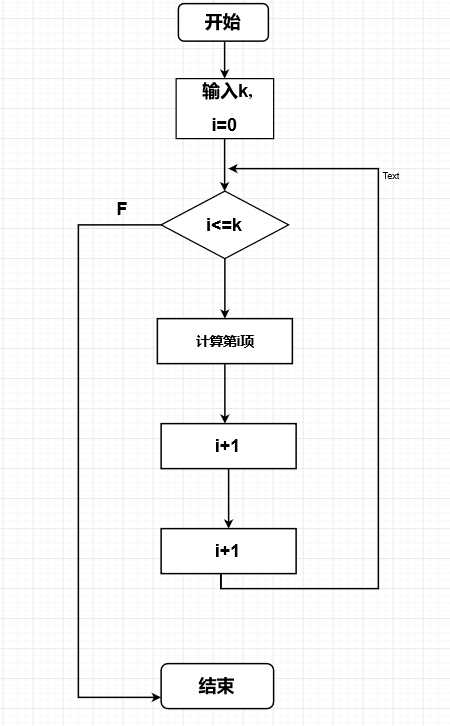?实验代码：

``` 1 #include<stdio.h>
2 #include<conio.h>
3 main()
4 {
5     int i,k,s=0;
6     printf("Enter a number:");
7     scanf("%d",&k);
8     for(i=1;i<=k;i++)
9     {
10         s=s+i;
11         printf("%d  ",s);
12
13     }
14  } ```

?问题：无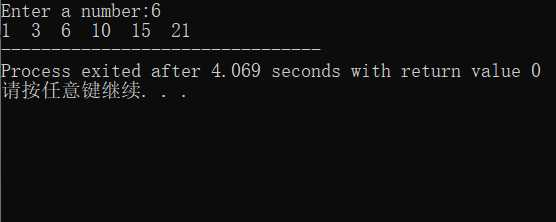?问题描述：编写一程序，求数列1，-3！，5！，-7！...(-1)^n-1(2n-1)前n项的和。n的值由键盘输入。

?要求：掌握for语句实现循环的方法。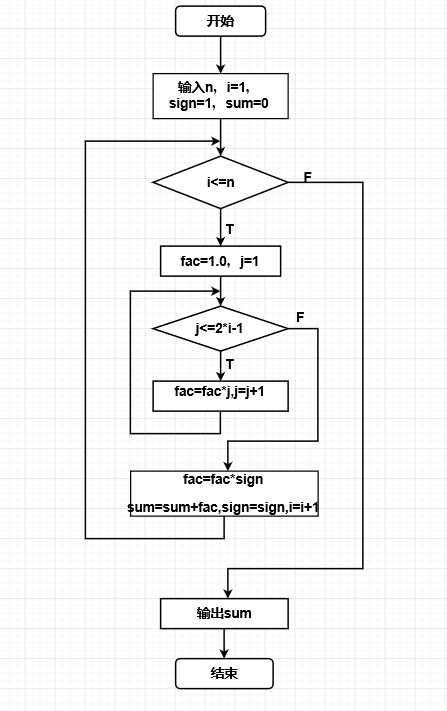?实验代码：

``` 1 #include<stdio.h>
2 main()
3 {
4     int n,i,j,sign=1;
5     float fac,sum;
6     printf("please input value of n:");
7     scanf("%d",&n);
8     sum=0.0;
9     for(i=1;i<=2*n-1;i=i+2)
10     {
11         fac=1.0;
12         for(j=1;j<=i;j++)
13         {
14             fac=fac*j;
15         }
16         fac=fac*sign;
17         sum=sum+fac;
18         sign=-sign;
19     }
20     printf("sum=%.0f",sum);
21 }```

?问题：太过于迷信书上的东西了，书上的流程图上（即上图）有一个错误，j<=2*i-1应该为j<=2*n-1;然而我一直没有发现，试了无数遍输出结果就是有错误。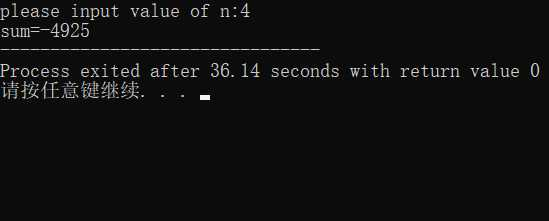?问题描述：从键盘上输入若干学生的成绩，统计并输出最高成绩和最低成绩，当输入负数时结束输入。

?要求：掌握while语句实验循环的方法。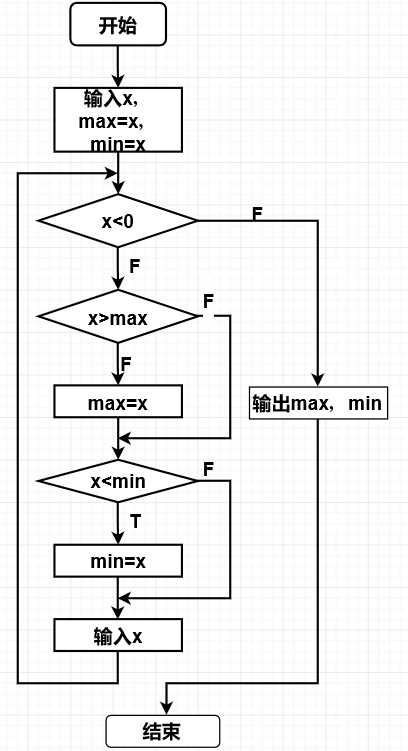?实验代码：

``` 1 #include<stdio.h>
2 #include<conio.h>
3 main()
4 {
5     float x,max,min;
6     printf("please input scores:");
7     scanf("%f",&x);
8     max=min=x;
9     while(x>0)
10     {
11         if(x>max) max=x;
12         if(x<min) min=x;
13         scanf("%f",&x);
14     }
15     printf("\nmax=%f\nmin=%f\n",max,min);
16  } ```

问题：刚开始没看明白这道题是什么意思，输入的是一串数而不是一个数。然后利用while语句比较大小，输出最大最小值。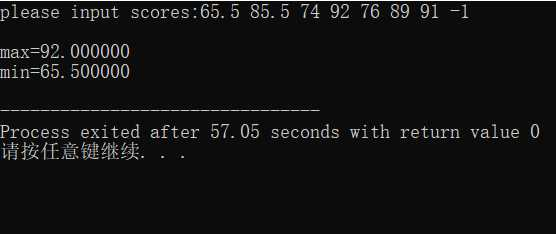?问题描述：求所有水仙花数（水仙花数是一个3位数的自然数，该数各位数的立方和等于该数本身，如153为水仙花数1^3+5^3+3^3=153）。

?要求：进一步掌握while语句实现循环的方法。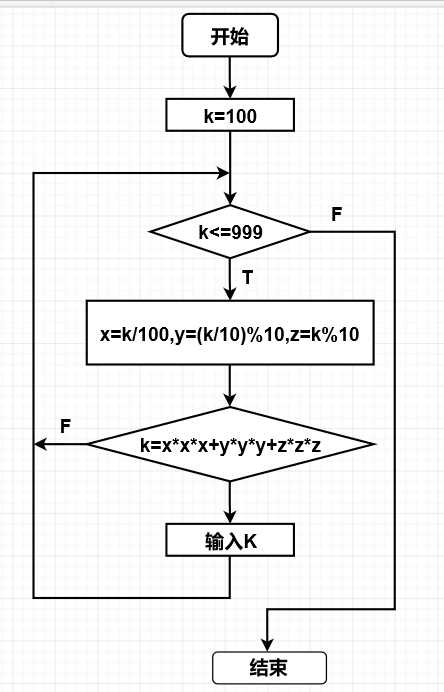?实验代码：

``` 1 #include<stdio.h>
2 main()
3 {
4     int x,y,z;
5     int k=100;
6     while(k>=100&&k<1000)
7     {
8         x=k/100;
9         y=(k/10)%10;
10         z=k%10;
11         if(k==x*x*x+y*y*y+z*z*z)
12         printf("%d\n",k);
13         k++;
14     }
15  } ```

问题：注意一下各个位数的提取就基本没问题。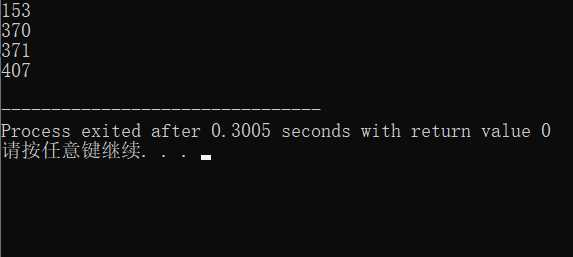?问题描述：求满足下列不等式求满足1+1/2+1/3+...+1/n>value这个不等式的n最小值，的n的最小值，其中，value是大于1的任何数。

?要求：掌握do-while语句实现循环的方法。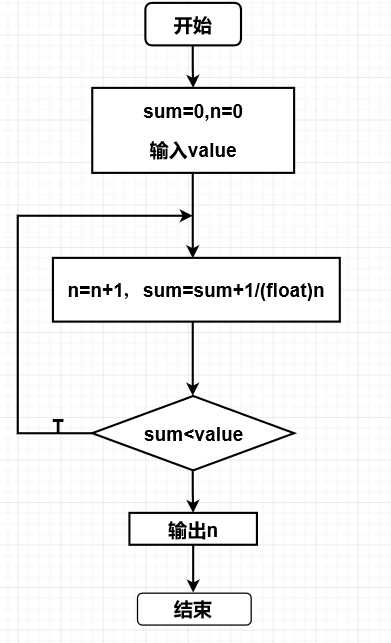?实验代码：

``` 1 #include<stdio.h>
2 main()
3 {
4     float sum,value;
5     int n;
6     printf("Input value:");
7     scanf("%f",&value);
8     sum=0.0;
9     n=0;
10     do
11     {
12         ++n;
13         sum+=1.0/(float)n;
14     }
15     while(sum<value);
16     printf("n=%d",n);
17 }```

问题：程序接收不等于右边的数值，因为是大于1的任何数，因此用float型定义。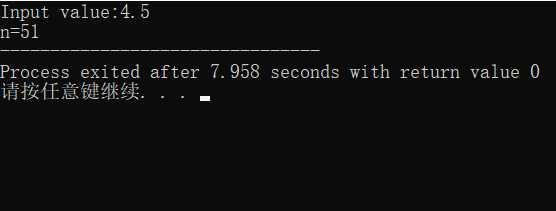?问题描述：输入4个数字字符，并把其转换为4位十进制整数后显示出来。

?要求：掌握while语句和for语句配合实现循环的方法。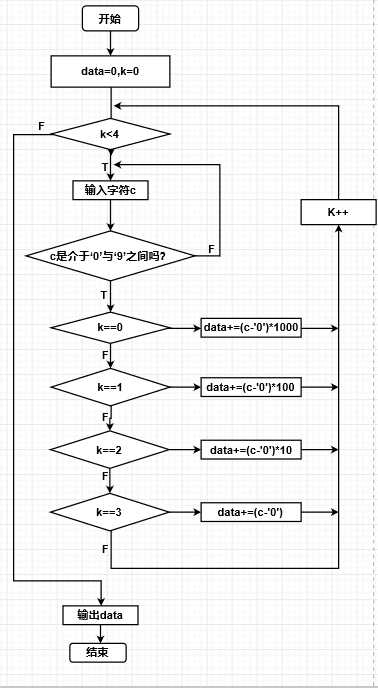?实验代码：

``` 1 #include<stdio.h>
2 main()
3 {
4     int data=0,k;
5     char c;
6     for(k=0;k<4;k++)
7     {
8         while(1)
9         {
10             c=getchar();
11             if(c>=‘0‘&&c<=‘9‘)
12             break;
13         }
14         if(k==0) data+=(c-‘0‘)*1000;
15         else if(k==1) data+=(c-‘0‘)*100;
16         else if(k==2) data+=(c-‘0‘)*10;
17         else if(k==3) data+=(c-‘0‘);
18     }
19     printf("Data=%d",data);
20 }```

问题：if语句内用“==”才会输出最后正确结果。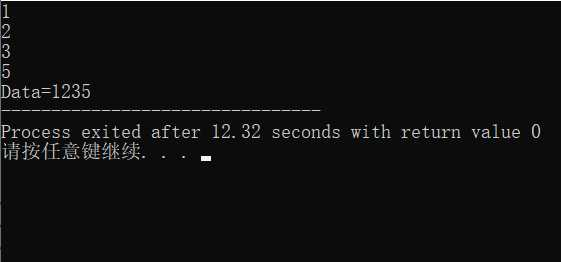?问题描述：有100匹马，要驮100担货物，其中，1匹大马可以驼3担，1匹中马可以驼两担，两匹小马可以驼一担，请问，大马、中马和小马可以有多少种组合。

?要求：掌握for语句嵌套实现循环的方法。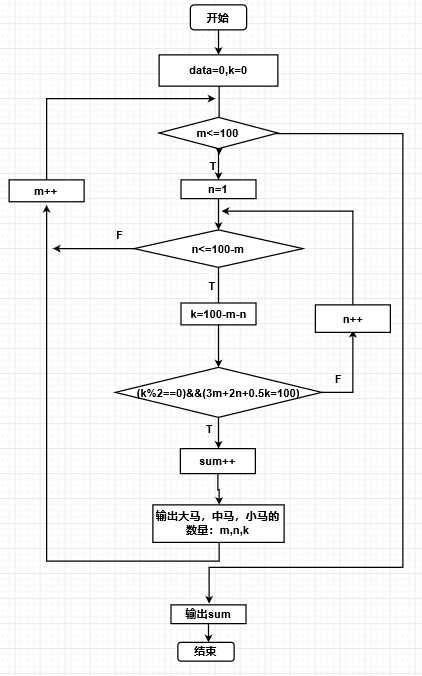?实验代码：

``` 1 #include<stdio.h>
2 main()
3 {
4     int m,n,k;
5     int sum=0;
6     printf("各种驮法如下:\n");
7     for(m=1;m<=100;m++)
8     {
9         for(n=1;n<=100-m;n++)
10         {
11             k=100-m-n;
12             if((k%2==0)&&(3*m+2*n+k/2==100))
13             {
14                 printf("大马%d匹；中马%d匹；小马%d匹.\n",m,n,k);
15                 sum++;
16             }
17         }
18     }
19     printf("共有%d种驮法",sum);
20 }```

问题：无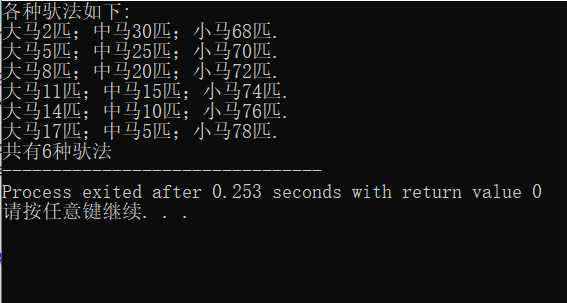?问题描述：编写程序，求一正整数等差数列的前6项的和，该数列前4项之和是26，前4项之积是880。

?要求：掌握for语句嵌套（3层）实现循环的方法，掌握continue的用法。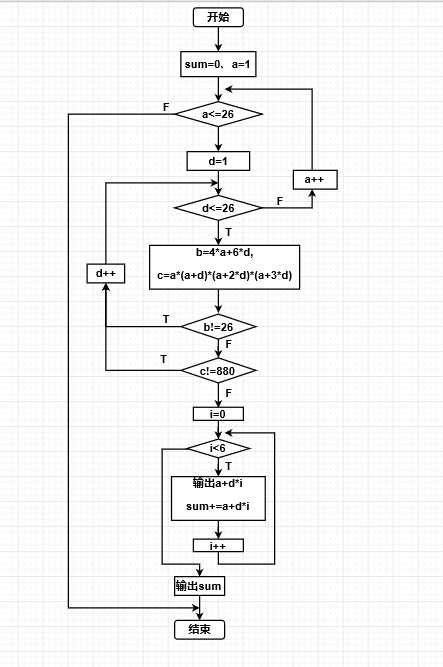?实验代码：

``` 1 #include<stdio.h>
2 main()
3 {
4     int a,b,c,d,i,sum=0;//a为首项，d为方差 ，b为前4项的和，c为前4项的积，第n项即为第i+1项；
5     for(a=1;a<=26;a++)
6     {
7         for(d=1;d<=26;d++)
8         {
9             b=4*a+6*d;
10             if(b!=26)
11                 continue;
12             else
13                 c=a*(a+d)*(a+2*d)*(a+3*d);
14             if(c!=880)
15                 continue;
16             else
17                 for(i=0;i<6;i++)
18                 {
19                     printf("%d,",a+i*d);
20                     sum=sum+(a+i*d);
21                 }
22         }
23     }
24     printf("\n数列的前六项和为:%d\n",sum);
25 }```

问题：利用for循环求解的时候，需要同时满足数列前4项值之和为26并且数列前4项之积为880。这部分需要注意一下continue语句。然后养成写注释的习惯（上面代码第4行），不然很容易搞混。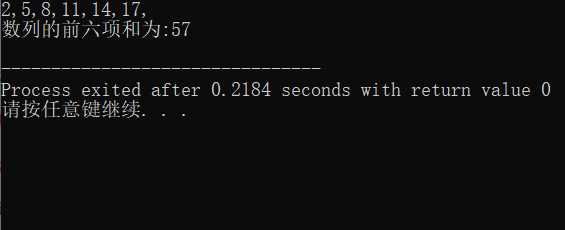?问题描述：有30个学生一起买小吃，共花钱50元，其中，每个大学生花3元，每个中学生花2元，每个小学生花1元，问大、中、小学生的人数分配共有多少种不同的解（去掉某类学生数为0的解）？

?要求：掌握for语句嵌套实现循环的方法，掌握continue的用法。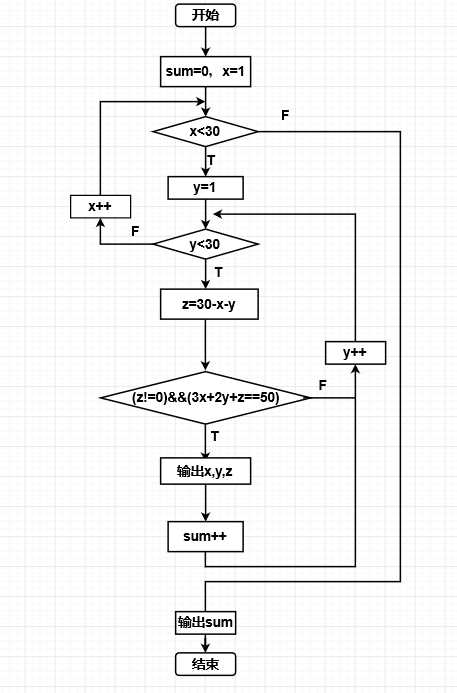?实验代码：

``` 1 #include<stdio.h>
2 main()
3 {
4     int x,y,z,sum;
5     sum=0;
6     for(x=1;x<30;x++)
7     {
8         for(y=1;y<30;y++)
9         {
10             z=30-x-y;
11             if(3*x+2*y+z==50)
12             {
13                 printf("大学生%d元\t中学生%d元\t小学生%d元\n",x,y,z);
14                 sum=sum+1;
15             }
16             else
17                 continue;
18         }
19     }
20     printf("\n共有%d种分钱方法\n",sum);
21 } ```

问题：无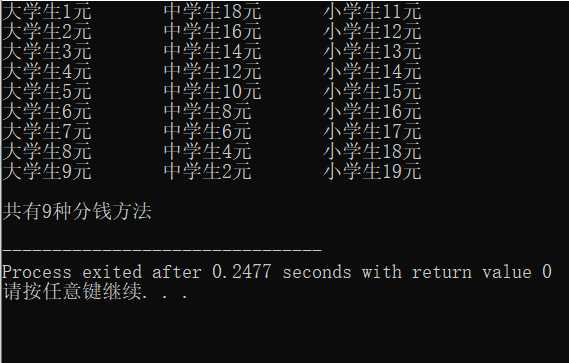-----------------------------------------------------------这是一条分割线----------------------------------------------------

(0)
(0)

© 2014 bubuko.com 版权所有 鲁ICP备09046678号-4